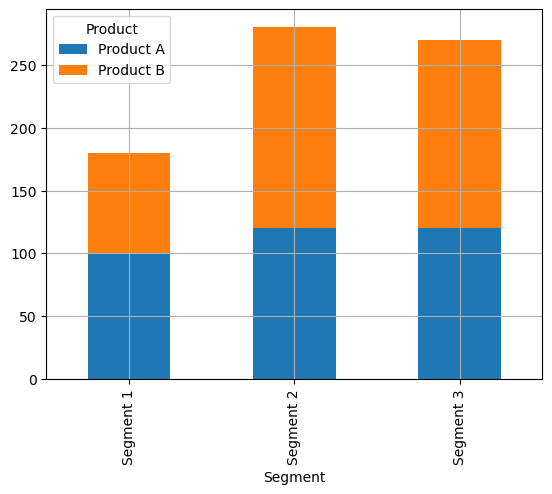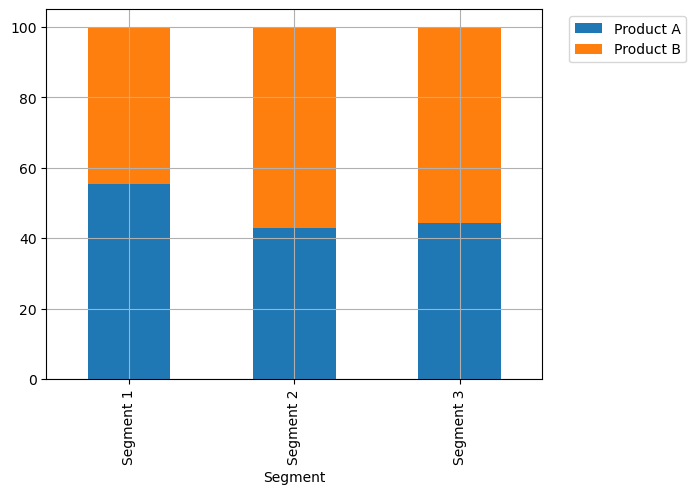A barplot is a graphical representation of data points in a dataset, where individual data points are represented by rectangular bars on a two-dimensional coordinate system. This type of plot allows us to visualize the distribution of categorical data by showing the frequency or count of each category along the plot.
Pandas, a powerful data manipulation library in Python, allow us to create easily barplots: check this introduction to barplots with pandas. In this post, we will explore how to leverage `Pandas` to create a stacked barplot.

## Libraries

Pandas is a popular open-source Python library used for data manipulation and analysis. It provides data structures and functions that make working with structured data, such as tabular data (like `Excel` spreadsheets or `SQL` tables), easy and intuitive.

To install Pandas, you can use the following command in your command-line interface (such as `Terminal` or `Command Prompt`):

`pip install pandas`

Matplotlib functionalities have been integrated into the pandas library, facilitating their use with `dataframes` and `series`. For this reason, you might also need to import the matplotlib library when building charts with Pandas.

This also means that they use the same functions, and if you already know Matplotlib, you'll have no trouble learning plots with Pandas.

``````import pandas as pd
import matplotlib.pyplot as plt``````

## Dataset

In order to create graphics with Pandas, we need to use pandas objects: `Dataframes` and `Series`. A dataframe can be seen as an `Excel` table, and a series as a `column` in that table. This means that we must systematically convert our data into a format used by pandas.

We generate 3 variables: 2 quantitative using `np.random.uniform()` and `np.random.normal()` functions and one qualitative, whose values depend on the values of the first qualitative variable.

``````data = {
"Product": ["Product A", "Product A", "Product A", "Product B", "Product B", "Product B"],
"Segment": ["Segment 1", "Segment 2", "Segment 3", "Segment 1", "Segment 2", "Segment 3"],
"Amount_sold": [100, 120, 120, 80, 160, 150]
}

df = pd.DataFrame(data)``````

## Simple stacked barplot

Once we've opened our dataset, we'll now create the graph.

This dataset represents sales data for different products (`Product A` and `Product B`) across various segments (`Segment 1`, `Segment 2`, and `Segment`3). The `"Amount_sold"` column represents the quantity of each product sold within each segment.

The `pivot()` function is used in this context to reshape the original DataFrame into a format suitable for creating a grouped barplot. In a grouped barplot, you typically want each category (in this case, each `product`) to have its own set of bars grouped by another categorical variable (in this case, the `segments`).

Then, we put `stacked=True` in order to specify that we want the bars on top of each others

``````# Pivot the data to have 'Product' as columns and 'Segment' as the index
pivot_df = df.pivot(index='Segment',
columns='Product',
values='Amount_sold')

# Create a grouped barplot
pivot_df.plot.bar(stacked=True,
grid=True)

plt.show()``````## Stacked barplot 100%

In order to make the graph 100% stacked, we have to modify the original dataset so that everything has the same scale and the sum faces 100%. We use the `div()` function from pandas.

``````# Pivot the data to have 'Product' as columns and 'Segment' as the index
pivot_df = df.pivot(index='Segment',
columns='Product',
values='Amount_sold')

# New dataframe with values on a 100% scale
pivot_df_percentage = pivot_df.div(pivot_df.sum(axis=1), axis=0) * 100

# Create a grouped barplot
pivot_df_percentage.plot.bar(stacked=True,
grid=True)

plt.legend(bbox_to_anchor=(1.04, 1), # shift the legend 4% on the right
loc='upper left')

# Display the plot
plt.show()``````## Going further

This post explains how to create a stacked barplot with pandas.

For more examples of how to create or customize your barplots, see the barplot section. You may also be interested in how to customize your barplot with pandas.

## Contact & Edit

👋 This document is a work by Yan Holtz. You can contribute on github, send me a feedback on twitter or subscribe to the newsletter to know when new examples are published! 🔥